Anzeige

# SECTION IV - CHAPTER 29 - Implied Volatility Basics

23. Mar 2023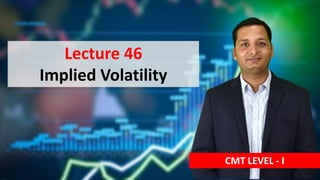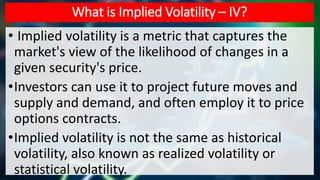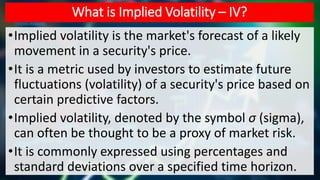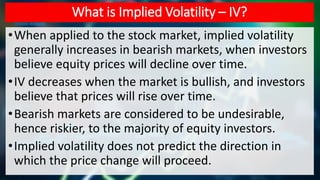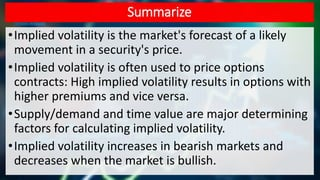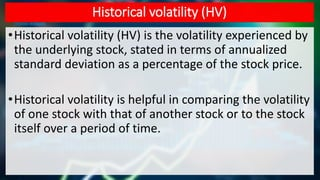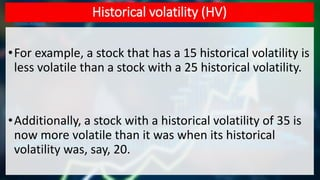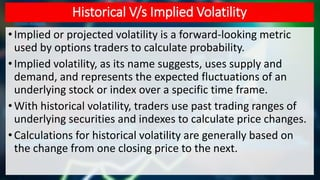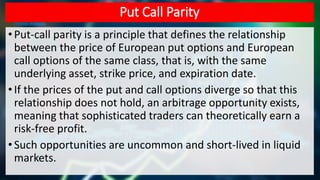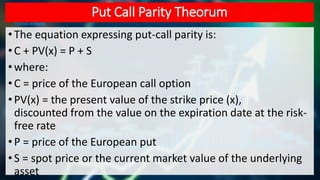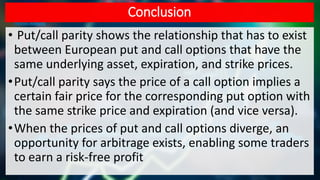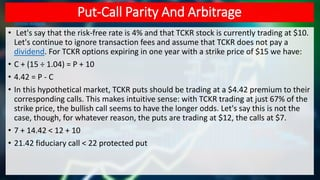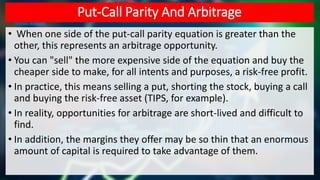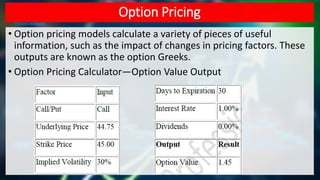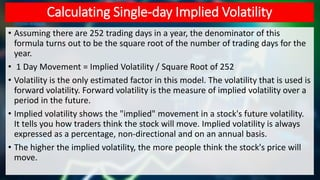1 von 15
Anzeige

### SECTION IV - CHAPTER 29 - Implied Volatility Basics

1. Lecture 46 Implied Volatility CMT LEVEL - I
2. What is Implied Volatility – IV? • Implied volatility is a metric that captures the market's view of the likelihood of changes in a given security's price. •Investors can use it to project future moves and supply and demand, and often employ it to price options contracts. •Implied volatility is not the same as historical volatility, also known as realized volatility or statistical volatility.
3. What is Implied Volatility – IV? •Implied volatility is the market's forecast of a likely movement in a security's price. •It is a metric used by investors to estimate future fluctuations (volatility) of a security's price based on certain predictive factors. •Implied volatility, denoted by the symbol σ (sigma), can often be thought to be a proxy of market risk. •It is commonly expressed using percentages and standard deviations over a specified time horizon.
4. What is Implied Volatility – IV? •When applied to the stock market, implied volatility generally increases in bearish markets, when investors believe equity prices will decline over time. •IV decreases when the market is bullish, and investors believe that prices will rise over time. •Bearish markets are considered to be undesirable, hence riskier, to the majority of equity investors. •Implied volatility does not predict the direction in which the price change will proceed.
5. Summarize •Implied volatility is the market's forecast of a likely movement in a security's price. •Implied volatility is often used to price options contracts: High implied volatility results in options with higher premiums and vice versa. •Supply/demand and time value are major determining factors for calculating implied volatility. •Implied volatility increases in bearish markets and decreases when the market is bullish.
6. Historical volatility (HV) •Historical volatility (HV) is the volatility experienced by the underlying stock, stated in terms of annualized standard deviation as a percentage of the stock price. •Historical volatility is helpful in comparing the volatility of one stock with that of another stock or to the stock itself over a period of time.
7. Historical volatility (HV) •For example, a stock that has a 15 historical volatility is less volatile than a stock with a 25 historical volatility. •Additionally, a stock with a historical volatility of 35 is now more volatile than it was when its historical volatility was, say, 20.
8. Historical V/s Implied Volatility • Implied or projected volatility is a forward-looking metric used by options traders to calculate probability. • Implied volatility, as its name suggests, uses supply and demand, and represents the expected fluctuations of an underlying stock or index over a specific time frame. • With historical volatility, traders use past trading ranges of underlying securities and indexes to calculate price changes. •Calculations for historical volatility are generally based on the change from one closing price to the next.
9. Put Call Parity • Put-call parity is a principle that defines the relationship between the price of European put options and European call options of the same class, that is, with the same underlying asset, strike price, and expiration date. •If the prices of the put and call options diverge so that this relationship does not hold, an arbitrage opportunity exists, meaning that sophisticated traders can theoretically earn a risk-free profit. • Such opportunities are uncommon and short-lived in liquid markets.
10. Put Call Parity Theorum • The equation expressing put-call parity is: •C + PV(x) = P + S • where: • C = price of the European call option • PV(x) = the present value of the strike price (x), discounted from the value on the expiration date at the risk- free rate • P = price of the European put • S = spot price or the current market value of the underlying asset
11. Conclusion • Put/call parity shows the relationship that has to exist between European put and call options that have the same underlying asset, expiration, and strike prices. •Put/call parity says the price of a call option implies a certain fair price for the corresponding put option with the same strike price and expiration (and vice versa). •When the prices of put and call options diverge, an opportunity for arbitrage exists, enabling some traders to earn a risk-free profit
12. Put-Call Parity And Arbitrage • Let's say that the risk-free rate is 4% and that TCKR stock is currently trading at \$10. Let's continue to ignore transaction fees and assume that TCKR does not pay a dividend. For TCKR options expiring in one year with a strike price of \$15 we have: • C + (15 ÷ 1.04) = P + 10 • 4.42 = P - C • In this hypothetical market, TCKR puts should be trading at a \$4.42 premium to their corresponding calls. This makes intuitive sense: with TCKR trading at just 67% of the strike price, the bullish call seems to have the longer odds. Let's say this is not the case, though, for whatever reason, the puts are trading at \$12, the calls at \$7. • 7 + 14.42 < 12 + 10 • 21.42 fiduciary call < 22 protected put
13. Put-Call Parity And Arbitrage • When one side of the put-call parity equation is greater than the other, this represents an arbitrage opportunity. • You can "sell" the more expensive side of the equation and buy the cheaper side to make, for all intents and purposes, a risk-free profit. • In practice, this means selling a put, shorting the stock, buying a call and buying the risk-free asset (TIPS, for example). • In reality, opportunities for arbitrage are short-lived and difficult to find. • In addition, the margins they offer may be so thin that an enormous amount of capital is required to take advantage of them.
14. Option Pricing • Option pricing models calculate a variety of pieces of useful information, such as the impact of changes in pricing factors. These outputs are known as the option Greeks. • Option Pricing Calculator—Option Value Output
15. Calculating Single-day Implied Volatility • Assuming there are 252 trading days in a year, the denominator of this formula turns out to be the square root of the number of trading days for the year. • 1 Day Movement = Implied Volatility / Square Root of 252 • Volatility is the only estimated factor in this model. The volatility that is used is forward volatility. Forward volatility is the measure of implied volatility over a period in the future. • Implied volatility shows the "implied" movement in a stock's future volatility. It tells you how traders think the stock will move. Implied volatility is always expressed as a percentage, non-directional and on an annual basis. • The higher the implied volatility, the more people think the stock's price will move.
Anzeige